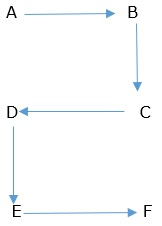# C program to represent the alphabets in spiral pattern

The spiral pattern to represent the alphabets is as follows −The logic used to represent the alphabets in spiral model is as follows −

if(rows<=13 && rows>=1){
for(i=1;i<=rows*2;i+=2){
if(k%2==1){
printf("%c %c",i+64,i+65);
k++;
}else{
printf("%c %c",i+65,i+64);
k++;
}
printf("");
}
}
else{
printf("Please Enter from 1 to 13 only");
}

## Program

Following is the C program to represent the alphabets in spiral pattern −

Live Demo

#include<stdio.h>
main(){
int i,rows,k=1;
printf("Enter number of Rows for Spiral Alpha Pattern from 1 to 13");
scanf("%d",&rows);
if(rows<=13 && rows>=1){
for(i=1;i<=rows*2;i+=2){
if(k%2==1){
printf("%c %c",i+64,i+65);
k++;
}else{
printf("%c %c",i+65,i+64);
k++;
}
printf("");
}
}else{
printf("Please Enter from 1 to 13 only");
}
}

## Output

When the above program is executed, it produces the following result −

Enter number of Rows for Spiral Alpha Pattern from 1 to 13
10
A B
D C
E F
H G
I J
L K
M N
P O
Q R
T S